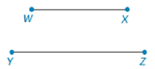Chapter 7.1, Problem 46EElementary Geometry For College St...

7th Edition
Alexander + 2 others
ISBN: 9781337614085

Solutions

Chapter
SectionElementary Geometry For College St...

7th Edition
Alexander + 2 others
ISBN: 9781337614085
Textbook Problem

Note: Exercises preceded by an asterisk are of a more challenging nature.In Exercises 39 and 42, refer to the line segments shown.Use the following theorem to construct the geometric mean of the numerical lengths of the segments W X - and Y Z - .Theorem: The length of the altitude to the hypotenuse of a right triangle is the geometric mean between the lengths of the segments of the hypotenuse.To determine

To construct:

The geometric mean of the numerical lengths of the segments WX- and YZ-.

Explanation

Theorem:

Geometric mean theorem:

The length of the altitude to the hypotenuse of a right triangle is the geometric mean between the lengths of the segments of the hypotenuse.

Calculation:

Consider a right angle triangle WXZ which consists of two right triangles WXY and WYZ as shown in figure.

Consider the right triangles WXY and WXZ.

XYWX=WXXZ

Consider the right triangles WXY and WYZ.

XYWY=WYWZ

Consider the right triangles WYZ and WXZ.

WZWY=XZWZ

From the above, we find that the length of the altitude to the hypotenuse of a right triangle is the geometric mean between the lengths of the segments of the hypotenuse

Still sussing out bartleby?

Check out a sample textbook solution.

See a sample solution

The Solution to Your Study Problems

Bartleby provides explanations to thousands of textbook problems written by our experts, many with advanced degrees!

Get Started

Prove that limx0+lnx=

Single Variable Calculus: Early Transcendentals, Volume I

In Exercises 1728, use the logarithm identities to obtain the missing quantity.

Finite Mathematics and Applied Calculus (MindTap Course List)

In Exercises 58. evaluate the expression. 5. |5 + 7| + |2|

Applied Calculus for the Managerial, Life, and Social Sciences: A Brief Approach

True or False: A trigonometric substitution is necessary to evaluate xx2+10dx.

Study Guide for Stewart's Single Variable Calculus: Early Transcendentals, 8th